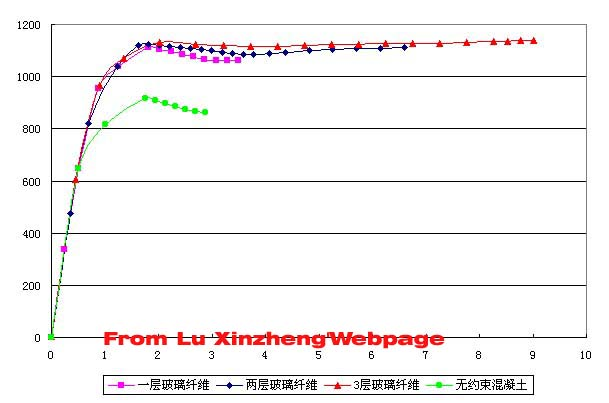FEA for FRP Confined Concrete Columns Paper download Finite Element Analysis of FRP Confined Square Columns under Uniaxial Loading China Civil Engineering Journal. 2003.36(2).46-51. Abstract: Fiber Reinforced Plastic (FRP) confined square columns under uniaxial loading are analyzed using finite element method (FEM). Compared with test results, the FEM results can effectively simulate the behavior of FRP confined square columns with proper numerical model. The mechanical mechanism of the specimens and the interaction between FRP material and concrete were analyzed based on the numerical results, which can be used for further research on numerical test and parameter analysis. Keywords: Fiber Reinforced Plastic, Strengthening, finite element analysis, confined concrete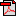Download Here Nonlinear finite element analysis for the FRP confined concrete square columns with ANSYS. Case: 1 Confined with Glass Fiber (GFRP) (Fiber Thickness: 0.3mm, Elastic Modulus: 100GPa, Concrete Strength: fc=28MPa, Poisson Ratio: 0.2)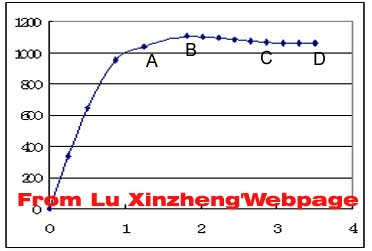Case: 2 Confined with Glass Fiber (GFRP) (Fiber Thickness: 0.6mm, Elastic Modulus: 100GPa, Concrete Strength: fc=28MPa, Poisson Ratio: 0.2)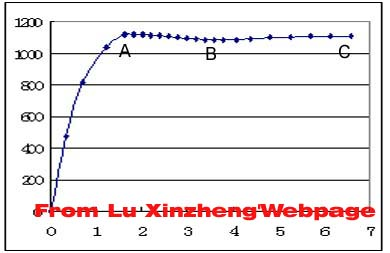Case: 3 Confined with Glass Fiber (GFRP) (Fiber Thickness: 0.9mm, Elastic Modulus: 100GPa, Concrete Strength: fc=28MPa, Poisson Ratio: 0.2)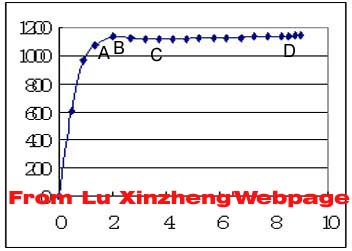Comparison